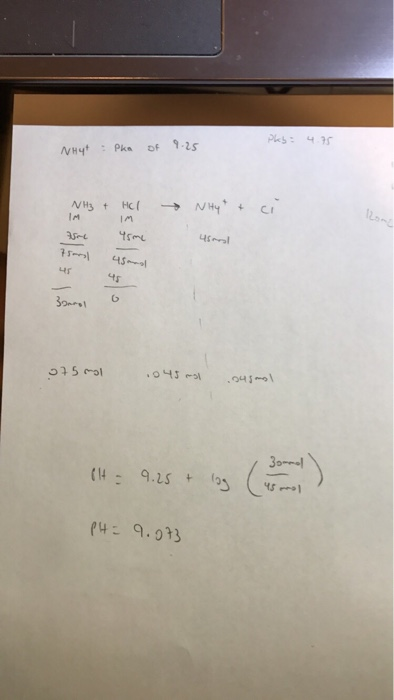# Question & Answer: Ammonium has a pka of 9.25. 45mL of 1.00 M HCl is added to 75 mL of a 1.00 M buffer…..

Ammonium has a pka of 9.25. 45mL of 1.00 M HCl is added to 75 mL of a 1.00 M buffer solution of this ammonium at PH 10. The resulting PH is?

Not sure what I’m doing wrong. Plz helpso I got your mistake you are not considering here that initially the ph of the solution is 10 .

Don't use plagiarized sources. Get Your Custom Essay on
Question & Answer: Ammonium has a pka of 9.25. 45mL of 1.00 M HCl is added to 75 mL of a 1.00 M buffer…..
GET AN ESSAY WRITTEN FOR YOU FROM AS LOW AS \$13/PAGE

so if we consider this then

Before adding hcl solution 10 = 9.25 + log [NH3]/[NH4+]

[NH3]/[NH4+] = 5.6234    -(1)

Also moles of (NH3 + NH4+) = 1M x 75 ml = 75 mmoles      -(2)

=> on solving equation 1 and equation2 we get

moles of NH3 were 63.676533 mmoles

moles of NH4+ were 11.323466 mmoles

after adding the HCL solution , 45 mmoles of H+ will react with NH3 to produce more NH4

according to equation

NH3 + H+   ————> NH4      moles of NH3 will react with H+ to produce more NH4+

hence final moles of NH3 will be 63.676533 – 45 = 18.676533 mmoles

also final moles of NH4+ will be 11.323466 + 45 = 56.3234 mmoles

now you should apply the equation which you have applied in your solution

ph = 9.25 + log [18.676533]/[56.3234] = 9.25 – 0.48 = 8.77 .